# Oops...

This website is made for modern browsers. You are seeing this, because your browser is missing a feature or two. Please install the latest update or switch to a modern browser. See you soon.

# Trim curves

Trimming curves implies that we split the curves and dump one of their remaining segments. Thus, we have to define the cutter and also, which side of the boundary contains the objects that will be disposed. The object can relate to the cutter in three ways:

1. The object is completely inside the trimming area.
2. The object has an intersection with the cutter.
3. The object is completely outside the trimming area.

The first step of trimming is to split all curves that have an intersection with the cutting shape, which is explained in the how-to Split curves. Then, we should combine all curves (cut and uncut) in a single data tree, which can be achieved with Combine Data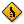Combine Data (Combine)
Sets  >  List  >  Combine Data
Combine non-null items out of several inputs
Inputs
Input 0 (0)Data to combine
Input 1 (1)Data to combine
Outputs
Result (R)Resulting data with as few nulls as possible
Index (I)Index of input that was copied into result
. Now, we eliminated the second possibility and all curves are either inside or outside our trimming area.

To check, whether a curve should be kept or disposed, we will retrieve its midpoint and then check if this point is inside or outside the trimming area. The midpoints are generated with Point On Curve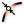Point On Curve (CurvePoint)
Curve  >  Analysis  >  Point On Curve
Evaluates a curve at a specific location
.
We could also use the component Curve Middle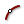Curve Middle (MidPt)
Curve  >  Analysis  >  Curve Middle
Get the point in the middle of a curve
Inputs
Curve (C)Curve for mid-point.
Outputs
Midpoint (M)Point in the middle of the curve
(new in Rhino 6.16).
We could also use the component Curve MiddleCurve Middle (MidPt)
Curve  >  Analysis  >  Curve Middle
Get the point in the middle of a curve
Inputs
Curve (C)Curve for mid-point.
Outputs
Midpoint (M)Point in the middle of the curve
(new in Rhino 6.16).
For the query, we need a list of Boolean values (True or False) which determine the state of each curve. How we get this list depends on the cutting objects:

### Trim curves with a plane

After splitting the curves with an XY Plane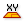XY Plane (XY)
Vector  >  Plane  >  XY Plane
World XY plane.
Inputs
Origin (O)Origin of plane
Outputs
Plane (P)World XY plane
and bundling them in a data tree, we use Point On CurvePoint On Curve (CurvePoint)
Curve  >  Analysis  >  Point On Curve
Evaluates a curve at a specific location
to retrieve their midpoints. By attaching a Deconstruct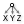Deconstruct (pDecon)
Vector  >  Point  >  Deconstruct
Deconstruct a point into its component parts.
Inputs
Point (P)Input point
Outputs
X component (X)Point {x} component
Y component (Y)Point {y} component
Z component (Z)Point {z} component
component to the midpoints, we can get the Z-coordinate. We then use Larger Than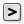Larger Than (Larger)
Maths  >  Operators  >  Larger Than
Larger than (or equal to)
Inputs
First Number (A)Number to test
Second Number (B)Number to test against
Outputs
Larger than (>)True if A > B
… or Equal to (>=)True if A >= B
to determine if the z-coordinate of the curve is above (True) or below (False) the Z-coordinate of the plane (here 0). We can use Dispatch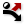Dispatch (Dispatch)
Sets  >  List  >  Dispatch
Dispatch the items in a list into two target lists.
Inputs
List (L)List to filter
Dispatch pattern (P)Dispatch pattern
Outputs
List A (A)Dispatch target for True values
List B (B)Dispatch target for False values
to sort the curves according to the list of Boolean values and, in this example, output A will contain all curves above our plane.

If we have an XZ Plane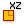XZ Plane (XZ)
Vector  >  Plane  >  XZ Plane
World XZ plane.
Inputs
Origin (O)Origin of plane
Outputs
Plane (P)World XZ plane
, a YZ Plane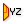YZ Plane (YZ)
Vector  >  Plane  >  YZ Plane
World YZ plane.
Inputs
Origin (O)Origin of plane
Outputs
Plane (P)World YZ plane
, or the origin of the plane is not at 0, we need to match this setup with the values we use for comparison on the components DeconstructDeconstruct (pDecon)
Vector  >  Point  >  Deconstruct
Deconstruct a point into its component parts.
Inputs
Point (P)Input point
Outputs
X component (X)Point {x} component
Y component (Y)Point {y} component
Z component (Z)Point {z} component
and Larger ThanLarger Than (Larger)
Maths  >  Operators  >  Larger Than
Larger than (or equal to)
Inputs
First Number (A)Number to test
Second Number (B)Number to test against
Outputs
Larger than (>)True if A > B
… or Equal to (>=)True if A >= B
.

If we use a construction plane that is tilted in space, we can use Project Point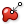Project Point (Project)
Vector  >  Point  >  Project Point
Project a point onto a collection of shapes
Inputs
Point (P)Point to project
Direction (D)Projection direction
Geometry (G)Geometry to project onto
Outputs
Point (P)Projected point
Index (I)Index of object that was projected onto
to project the midpoints onto the plane and set the projection direction D to the normal of the plane. Output I will now return 0 or -1 depending on whether a point hits the plane with the given direction. This output can be used with DispatchDispatch (Dispatch)
Sets  >  List  >  Dispatch
Dispatch the items in a list into two target lists.
Inputs
List (L)List to filter
Dispatch pattern (P)Dispatch pattern
Outputs
List A (A)Dispatch target for True values
List B (B)Dispatch target for False values
to separate the curves.

### Trim curves with a cutting curve

When we need to trim curves that are inside or outside a boundary curve, we can use a closed cutting curve (as in the schematic sketch at the beginning). Again we use Point On CurvePoint On Curve (CurvePoint)
Curve  >  Analysis  >  Point On Curve
Evaluates a curve at a specific location
to get the midpoints and then we check with Point In Curve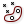Point In Curve (InCurve)
Curve  >  Analysis  >  Point In Curve
Test a point for closed curve containment.
Inputs
Point (P)Point for region inclusion test
Curve (C)Boundary region (closed curves only)
Outputs
Relationship (R)Point/Region relationship (0 = outside, 1 = coincident, 2 = inside)
Point (P')Point projected on region plane.
if the curves' midpoints are inside the boundary curve. Output R provides this information (0 = outside, 1 = coincident, 2 = inside) and we use Sift Pattern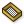Sift Pattern (Sift)
Sets  >  List  >  Sift Pattern
Sift elements in a list using a repeating index pattern.
Inputs
List (L)List to sift
Sift Pattern (P)Sifting pattern
Outputs
Output 0 (0)Output for sift index 0
Output 1 (1)Output for sift index 1
to sort accordingly (zoom in to add another output grip). Now output 0 contains all curves that are outside our cutting shape.

### Trim curves with a cutting solid

In cases where all curves are arbitrarily orientated in the three-dimensional space, we can use a solid as boundary for the trim. To check if a midpoint is inside a solid (here a Domain Box), we use Point In Brep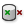Point In Brep (BrepInc)
Surface  >  Analysis  >  Point In Brep
Test whether a point is inside a closed brep
Inputs
Brep (B)Brep for inclusion test
Point (P)Point for inclusion test
Strict (S)If true, then the inclusion is strict
Outputs
Inside (I)True if point is on the inside of the Brep.
. A DispatchDispatch (Dispatch)
Sets  >  List  >  Dispatch
Dispatch the items in a list into two target lists.
Inputs
List (L)List to filter
Dispatch pattern (P)Dispatch pattern
Outputs
List A (A)Dispatch target for True values
List B (B)Dispatch target for False values
component will do the separation and we can then find all curves that are outside the solid at output B.

This page is open source. Edit it on GitHub or see how you can contribute.